# IF Function

Tests a given condition and returns one value for a TRUE result, and another for a FALSE result

## What is an Excel IF Statement?

The Excel IF Statement tests a given condition and returns one value for a TRUE result and another value for a FALSE result. For example, if sales total more than \$5,000, then return a “Yes” for Bonus – Otherwise, return a “No” for Bonus. We can also use the IF function to evaluate a single function, or we can include several IF functions in one formula. Multiple IF statements in Excel are known as nested IF statements.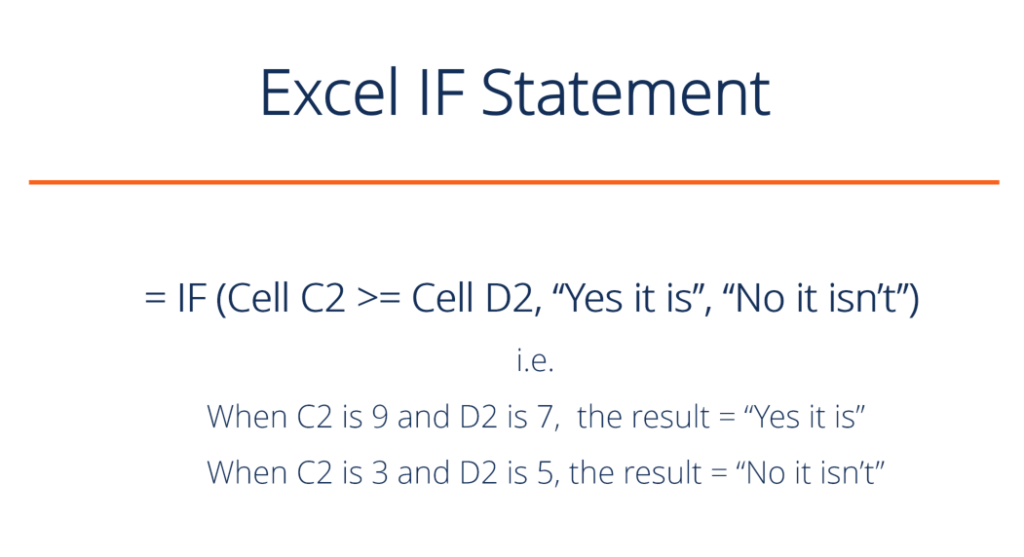As a financial analyst, the IF function is used often to evaluate and analyze data by evaluating specific conditions.

The function can be used to evaluate text, values, and even errors. It is not limited to only checking if one thing is equal to another and returning a single result. We can also use mathematical operators and perform additional calculations, depending on our criteria. We can also nest multiple IF functions together to perform multiple comparisons.

### IF Formula

=IF(logical_test, value_if_true, value_if_false)

The function uses the following arguments:

1. Logical_test (required argument) – This is the condition to be tested and evaluated as either TRUE or FALSE.
2. Value_if_true (optional argument) – The value that will be returned if the logical_test evaluates to TRUE.
3. Value_if_false (optional argument) – The value that will be returned if the logical_test evaluates to FALSE.

When using the IF function to construct a test, we can use the following logical operators:

• = (equal to)
• > (greater than)
• >= (greater than or equal to)
• < (less than)
• <= (less than or equal to)
• <> (not equal to)

### How to use the Excel IF Function

To understand the uses of the Excel IF statement function, let’s consider a few examples:

#### Example 1 – Simple Excel IF Statement

Suppose we wish to do a very simple test. We want to test if the value in cell C2 is greater than or equal to the value in cell D2. If the argument is true, then we want to return some text stating “Yes it is”, and if it’s not true, then we want to display “No it isn’t”.

You can see exactly how the Excel IF statement works in the simple example below.

Result when true: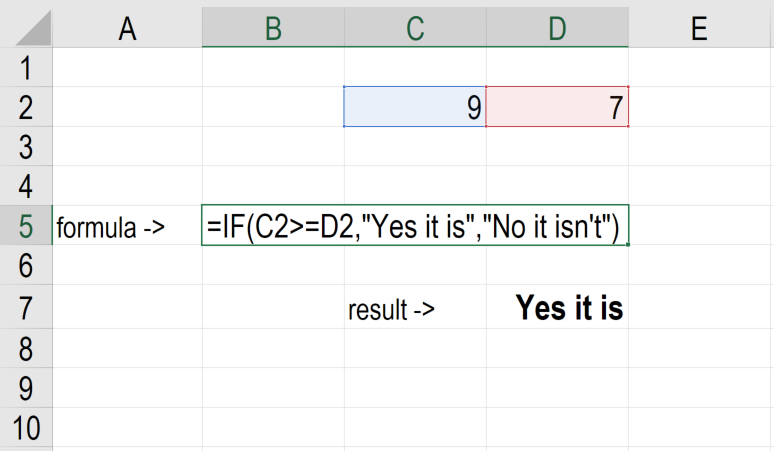Result when false: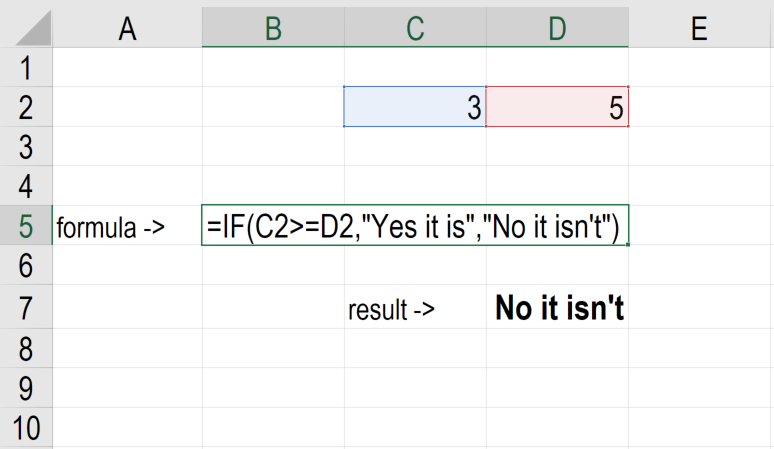#### Example 2 – Excel IF Statement

Suppose we wish to test a cell and ensure that an action is taken if the cell is not blank. We are given the data below: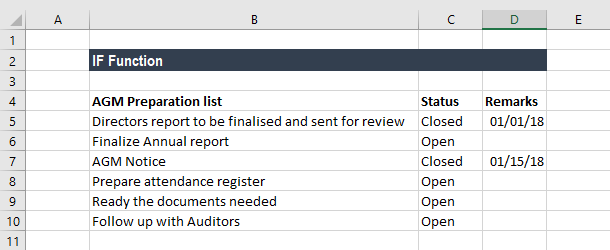In the worksheet above, we listed AGM-related tasks in Column A. Remarks contain the date of completion. In Column B, we will use a formula to check if the cells in Column C are empty or not. If a cell is blank, the formula will assign the status “open.” However, if a cell contains a date, then the formula will assign a status of “closed.” The formula used is: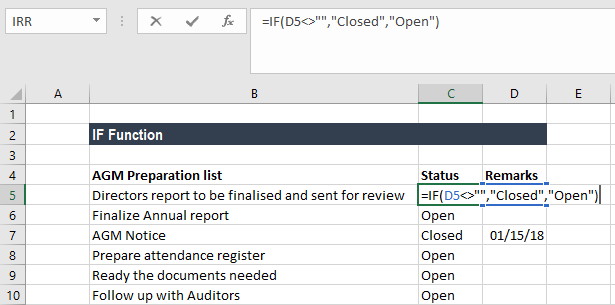We get the results below: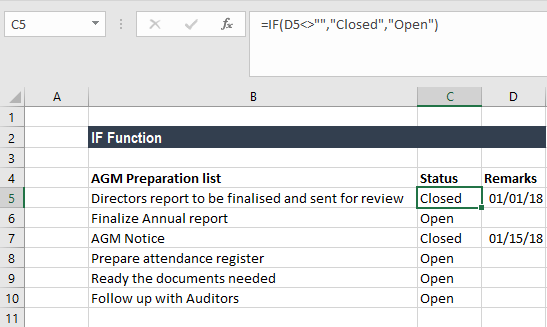#### Example 3 – Excel IF Statement

Sellers frequently provide a discount based on the quantity purchased. Suppose we are given the following data: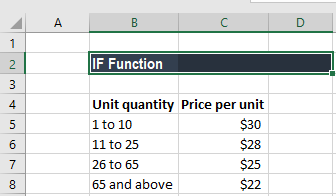Using multiple IF functions, we can create a formula to check multiple conditions and perform different calculations depending on what amount range the specified quantity falls in. To calculate the total price for 100 items, the formula will be: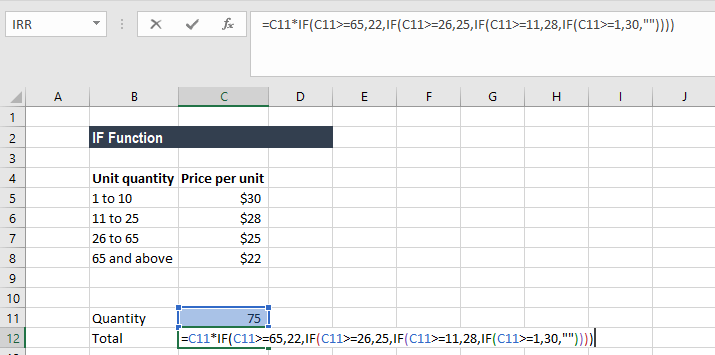We get the result below: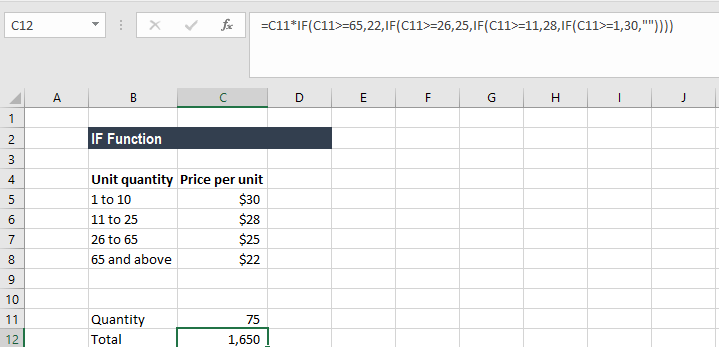### Things to remember about the IF Function

1. The Excel IF function will work if the logical_test returns a numeric value. In such a case, any non-zero value is treated as TRUE and zero is treated as FALSE.
2. #VALUE! error – Occurs when the given logical_test argument cannot be evaluated as TRUE or FALSE.
3. When any of the arguments are provided to the function as arrays, the IF function will evaluate every element of the array.
4. If we wish to count conditions, we should use the COUNTIF and COUNTIFS functions.
5. If we wish to add up conditions, we should use the SUMIF and SUMIFS functions.

### Reasons to use an Excel IF Statement

There are many reasons why an analyst or anyone who uses Excel would want to build IF formulas.

Common examples include:

• To test if an argument is true or false
• To output a NUMBER
• To output some TEXT
• To generate a conditional formula (e.g., the result is C3+B4 if true and N9-E5 if false)
• To create scenarios to be used in financial modeling
• To calculate a debt schedule or a fixed asset depreciation schedule in accounting

Thanks for reading CFI’s guide on how to use an Excel IF Statement. By taking the time to learn and master these functions, you’ll significantly speed up your financial analysis. To learn more, check out these additional CFI resources: